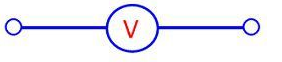Search
• Raj

# What is Voltmeter?

Updated: Jan 19, 2021

Definition:The instrument which measures the voltage or potential difference in volts is known as the voltmeter. It works on the principle that the torque is generated by the current which induces because of measurand voltage and this torque deflects the pointer of the instrument. The deflection of the pointer is directly proportional to the potential difference between the points. The voltmeter is always connected in parallel with the circuit.

### Symbolic Representation of voltmeter

The voltmeter is represented by the alphabet V inside the circle along with the two terminals.Why is Voltmeter connected in Parallel?

The voltmeter constructs in such a manner that their internal resistance always remains high. If it connects in series with the circuit, it minimises the current which flows because of the measurand voltage. Thus, disturb the reading of the voltmeter.The voltmeter always connects in parallel with the circuit so that the same voltage drop occurs across it. The high resistance of the voltmeter combines with the impedance of the element across which it is connected. And the overall impedance of the system is equal to the impedance that the element had. Thus, no obstruction occurs in the circuit because of the voltmeter, and the meter gives the correct reading.

## Why Voltmeter has High Resistance?

The voltmeter is constructed with very high internal resistance because it measures the potential difference between the two points of the circuit. The voltmeter does not change the current of the measuring device.

If the voltmeter has low resistance, the current passes through it, and the voltmeter gives the incorrect result. The high resistance of the voltmeter does not allow the current to pass through it and thus the correct reading is obtained.

### Types of Voltmeter

The voltmeter is classified into three ways. The classification of the voltmeter is shown in the figure below.On the basis of the construction, the voltmeter is of the following types.

PMMC Voltmeter

It works on the principle that the current carrying conductor placed in the magnetic field and because of the current the force acting on the conductor. The current induces in the PMMC instrument because of the measurand voltage, and this current deflects the pointer of the meter.

The PMMC voltmeter uses for DC measurement. The accuracy of the instrument is very high and having low power consumption. The only disadvantage of the instrument is that it is very costly. The range of the PMMC voltmeter increases by connecting the resistance in series with it.

MI Voltmeter

The MI instrument means moving iron instrument. This instrument uses for the measurement of both the AC and DC voltage. In this type of instrument, the deflection is directly proportional to the voltage of the coil. The moving iron instrument is classified into two types.

• Attraction Type Moving Iron Instrument

• Repulsion Type Moving Iron Instrument

Electro-dynamometer Voltmeter

The electro-dynamometer voltmeter is used for measuring the voltage of both AC and DC circuit. In this type of instruments, the calibration is same both for the AC and DC measurement.

Rectifier voltmeter

Such type of instrument is used in AC circuits for voltage measurement. The rectifier instrument converts the AC quantity into the DC quantity by the help of the rectifier. And then the DC signal is measured by the PMMC instrument.

The following are the classification of instruments regarding the displays of output reading.

Analogue Voltmeter

The analogue voltmeter uses for measuring the AC voltage. It displays the reading through the pointer which is fixed on the calibrated scale. The deflection of the pointer depends on the torque acting on it. The magnitude of the develops torque is directly proportional to the measuring voltage.

Digital Voltmeter

The voltmeter which displays the reading in the numeric form is known as the digital voltmeter. The digital voltmeter gives the accurate result.

The instrument which measures the direct current is known as the DC voltmeter, and the AC voltmeter is used in the AC circuit for alternating voltage measurement.